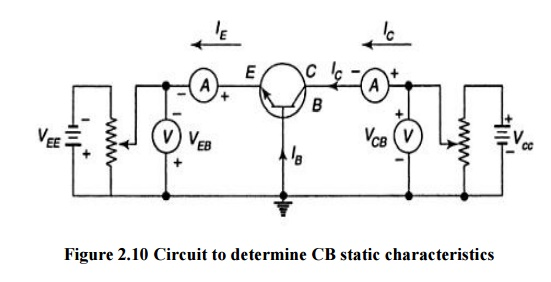Home | | Electronic Devices and Circuits | Configuration of Transistor Circuit: CB, CE, CC configuration Input and Output Characteristics

# Configuration of Transistor Circuit: CB, CE, CC configuration Input and Output Characteristics

Three types of configuration is available 1) Common base(CB) configuration 2) Common emitter (CE) configuration 3) Common collector (CC) configuration

CONFIGURATION OF TRANSISTOR CIRCUIT

A transistor is a three terminal device. But require '4‘ terminals for connecting it in a circuits.

(i.e.) 2 terminals for input, 2 terminals for output.

Hence one of the terminal is made common to the input and output circuits. Common terminal is grounded.

## Ø         TYPES OF CONFIGURATIONS

Three types of configuration is available

1)                    Common base(CB) configuration

2)                    Common emitter (CE) configuration

3)                    Common collector (CC) configuration

## 1. COMMON BASE(CB) CONFIGURATION

In common base configuration circuit is shown in figure. Here base is grounded and it is used as the common terminal for both input and output.It is also called as grounded base configuration. Emitter is used as a input terminal where as collector is the output terminal.

## Input characteristics:

It is defined as the characteristic curve drawn between input voltage to input current whereas output voltage is constant.

To determine input characteristics, the collector base voltage VCB is kept constant at zero and emitter current IE is increased from zero by increasing VEB.This is repeated for higher fixed values of VCB.

A curve is drawn between emitter current and emitter base voltage at constant collector base voltage is shown in figure 2.11.When VCB is zero EB junctions is forward biased. So it behaves as a diode so that emitter current increases rapidly.## Ø          Output Characteristics

It is defined as the characteristic curve drawn between output voltage to output current whereas input current is constant.To determine output characteristics, the emitter current IE is kept constant at zero and collector current Ic is increased from zero by increasing VCB.This is repeated for higher fixed values of IE.

From the characteristic it is seen that for a constant value of IE, Ic is independent of VCB and the curves are parallel to the axis of VCB.As the emitter base junction is forward biased the majority carriers that is electrons from the emitter region are injected into the base region.

In CB configuration a variation of the base-collector voltage results in a variation of the quasi- neutral width in the base. The gradient of the minority-carrier density in the base therefore changes, yielding an increased collector current as the collector-base current is increased. This effect is referred to as the Early effect.## 2. CE CONFIGURATION

In common emitter configuration circuit is shown in figure. Here emitter is grounded and it is used as the common terminal for both input and output. It is also called as grounded emitter configuration. Base is used as a input terminal whereas collector is the output terminal.## Ø          Input Characteristics

It is defined as the characteristic curve drawn between input voltages to input current whereas output voltage is constant.

To determine input characteristics, the collector base voltage VCB is kept constant at zero and base current IB is increased from zero by increasing VBE.This is repeated for higher fixed values of VCE.

A curve is drawn between base current and base emitter voltage at constant collector base voltage is shown in figure2.14. Here the base width decreases. So curve moves right as VCE increases.## Ø          Output Characteristics

It is defined as the characteristic curve drawn between output voltage to output current whereas input current is constant.

To determine output characteristics, the base current IB i s kept constant at zero and collector current Ic is increased from zero by increasing VCE.This is repeated for higher fixed values of IB.

From the characteristic it is seen that for a constant value of IB, Ic is independent of VCB and the curves are parallel to the axis of VCE.The output characteristic has 3 basic regions:

-         Active region –defined by the biasing arrangements.

-         Cutoff region – region where the collector current is 0A

-         Saturation region- region of the characteristics to the left of VCB = 0V.## 3. CC CONFIGURATION

In common collector configuration circuit is shown in figure. Here collector is grounded and it is used as the common terminal for both input and output. It is also called as grounded collector configuration. Base is used as a input terminal whereas emitter is the output terminal.## Ø        Input Characteristics

It is defined as the characteristic curve drawn between input voltage to input current whereas output voltage is constant.

To determine input characteristics, the emitter base voltage VEB is kept constant at zero and base current IB is increased from zero by increasing VBC.This is repeated for higher fixed values of VCE.A curve is drawn between base current and base emitter voltage at constant collector base voltage is shown in figure2.17.## Ø        Output Characteristics

It is defined as the characteristic curve drawn between output voltage to output current whereas input current is constant.

To determine output characteristics, the base current  IB  is kept constant at zero and emitter current IE is increased from zero by increasing VEC. This is repeated for higher fixed values of IB.

From the characteristic it is seen that for a constant value of IB, IE is independent of VEB and the curves are parallel to the axis of VEC.A comparison of CB, CE and CC ConfigurationsStudy Material, Lecturing Notes, Assignment, Reference, Wiki description explanation, brief detail
Electronic Devices and Circuits : Transistors : Configuration of Transistor Circuit: CB, CE, CC configuration Input and Output Characteristics |